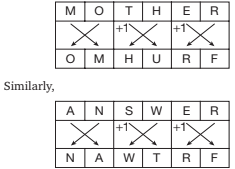# Mathematical Reasoning - Coding Decoding

>>>>>>>>Coding Decoding

• A

NFMWZMX• B

VMZWMFN• C

NFMWZMV• D

MIMXZMV• Option : C
• Explanation : Refer to Tables 5.1 and 5.2.
For example, C is ranked 3 in Table 5.1. The same rank denotes ‘X’ in Table 5.2. Thus, X is coded for C.
Thus, CERTAIN has been coded as XVIGZRM. Similarly, MUNDANE can be coded as NFMWZMV.

• A

TROFDER• B

FORTRED• C

TROFRED• D

FORTDER• Option : A
• Explanation : Here, the code is the reverse of the given word. The answer is TROFDER, which is the reverse of RED FORT.

• A

NBWRRF• B

MAVSPE• C

NBWTRD• D

NAWTRF• Option : D
• Explanation :• A

R F U O J S P• B

P F U O J S R• C

P S J O U F P• D

R S J O U F P• Option : A
• Explanation : The first and the last letters of the word swap their positions. All other letters are moved one place and get placed in the reverse manner.

• A

SU• B

SV• C

RV• D

RURelated Quiz.
Coding Decoding